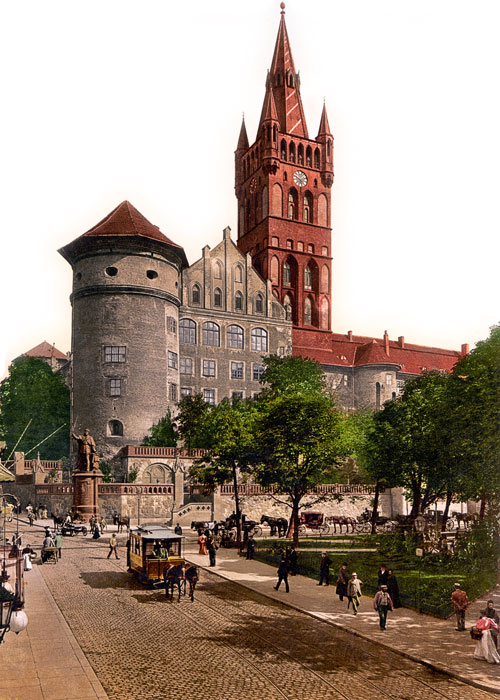## อภิธานศัพท์

เลือกหนึ่งในคำหลักทางด้านซ้าย ...

# Graphs and NetworksThe Bridges of Königsberg

เวลาอ่านหนังสือ: ~20 min

One of the first mathematicians to think about graphs and networks was Leonhard Euler. Euler was intrigued by an old problem regarding the town of Königsberg near the Baltic Sea.

The river Pregel divides Königsberg into four separate parts, which are connected by seven bridges. Is it possible to walk around the city crossing all of the bridges exactly once – but not more than once? (You can start and finish anywhere, not necessarily in the same place.)

Try to find a valid route by drawing on these maps:### Map 4

In the case of Königsberg it seems to be impossible to find a valid route, but some of the other cities do work. Euler managed to find a simple rule that can be applied to any city, without having to try lots of possibilities – using graph theory.

First, we need to convert the city maps into graphs with edges and vertices. Every island or region of land is represented by and every bridge connecting two regions is represented by a corresponding .

Now the problem of “touring a city while crossing every bridge exactly once” has become a problem of “drawing a graph with one continuous stroke while tracing every edge exactly once”.

On paper, come up with a few different graphs and then try to work out which ones can be drawn with a single, continuous stroke.

Just like for the city maps before, we find that some graphs are possible while others are not. To help us understand why, let us label every vertex with its degree. Then we can colour the vertices in different ways, and try to reveal a pattern:

Comparing these numbers for graphs which are possible, and those which are not possible, it seems that a graph can be drawn if it . This condition can be explained if we look at just a single vertex in the graph:

Here you can see a single, magnified vertex in a graph.

If we draw the graph, we will eventually have an edge leading towards this vertex, and then another one leading away. This makes two edges meeting at the vertex.

Maybe the vertex is a crossing rather than a corner. In that case there will be another edge leading towards the vertex, and another edge leading away. Now we have four edges.

And in some graphs, there may even be a third pair of edges leading towards and away from the vertex. Now there are six edges.

Notice that, either way, there always is an even number of edges meeting at the vertex.

The only two exceptions are the vertices where the path starts, and where it ends – these two may have an odd number of edges. If the start and end point are the same, all vertices in the graph are even.

If you scroll back to the map of Königsberg, you will find that there are more than two islands with an odd number of bridges. Therefore, a route that crosses every bridge exactly once is indeed impossible – and this is what Leonard Euler discovered.

Euler’s discovery may not seem particularly useful in real life, but graphs are at the foundation of many other geographic problems, such as finding directions between two locations. We will discover more of these applications later.

Archie